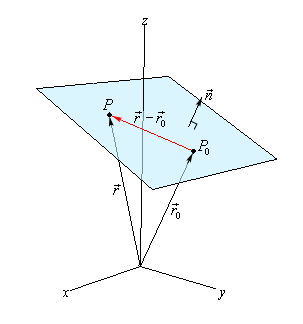## In Focus : 3D Geometry

The Coordinate Geometry is a vast subject in the field of Mathematics. The expanse of coordinate geometry is infinite as more and more dimensions are being discovered in the real world. However, we now limit our studies upto only three dimensions. This article will deal with all the basics to charge you up for 3D Geometry!

The topic of 3D geometry is quite important and a bit complicated as compared to its counterpart two dimensional geometry. One of the ways of describing a 3D object is by approximating or assuming its shape as a mesh of triangles. A triangle is generally defined by three vertices wherein the positions of the vertices are described by the coordinates x, y and z. The major heads that are included in 3D coordinate geometry are the direction ratios and direction cosines of a line segment along with definitions of the plane.

## Direction Cosines of a Line Segment

The direction cosines are the cosines of the angles between a line and the coordinate axis. If we have a vector (a, b, c) in three dimensional space, then the direction cosines of the vector are defined as

cos α = a/ √(a2 + b2 + c2), cos β = b/ √(a2 + b2 + c2), cos γ = c/ √(a2 + b2 + c2)## Direction Ratios

If l, m and n are the direction cosines then the direction ratios say a, b and c are given by

l = ± a/√ Σa2, m = ± b/√ Σa2, n = ± c/√ Σa2.

Points to remember:

• While the direction cosines of a line segment are always unique, the direction ratios are never unique and in fact they can be infinite in number.
• If the direction cosines of a line are l, m and n then they satisfy the relation l2 + m2 + n2 = 1.
• If the direction cosines of a line segment AB are (l, m, n) then those of line BA will be (-l, -m, -n).

## Angle Between Two Lines

Let us assume that θ is the angle between the two lines say AB and AC whose direction cosines are l1, m1 and n1 and l2, m2 and n2 then

cos θ = l1l2 + m1m2 + n1n2

Also if the direction ratios of two lines a1, b1 and c1 and a2, b2 and c2 then the angle between two lines is given by

cos θ = (a1a2 + b1b2 + c1c2)/ √(  a12 + b12 + c12) . √ (a22 + b22 + c22)

### Then what is the Condition for Parallel or Perpendicular Lines?

When the two lines are perpendicular, the angle between the lines is 90° which gives the condition of perpendicularity as

l1l2 + m1m2 + n1n2 = 0

or this implies a1a2 + b1b2 + c1c2 = 0.

Similarly, when the two lines are parallel, the angle between them i.e. θ = 0.

This gives l1/l2 = m1/m2 = n1/n2

This also gives a1/a2 = b1/b2 = c1/c2

## Projection of a line segment on a given line

Suppose we have a line segment joining the points P (x1, y1, z1) and Q(x2, y2, z2), then the projection of this line on another line having direction cosines as l, m, n is AB =  l(x2-x1) + m(y2-y1) + n(z2-z1).

## Plane

A plane is a flat, two-dimensional surface that extends infinitely far. A plane is the two-dimensional analogue of a point(zero dimensions), a line(one dimension) and three-dimensional space. A plane in three-dimensional space has the equation

ax + by + cz + d = 0

where at least one of the numbers and must be non-zero. A plane in the 3D coordinate space is determined by a point and a vector that is perpendicular to the plane.

A plane in the 3D coordinate space is determined by a point and a vector that is perpendicular to the plane. Let P0 = (xo,yo,zobe the point given, and n the orthogonal vector. Also, let P = (x,y,z) be any point in the 3D space, and and ro the position vectors of the points and Po respectively. Now, if we let n = (a,b,c)  then since Pois perpendicular to n we haveHope this article on 3D Geometry helps with your preparation. You can check out vectors here to help with your prep work. All the best!

Access 300,000+ questions curated by India’s top rankers.

+91
No thanks.

## Request a Free 60 minute counselling session at your home

Please enter a valid phone number
•7,829,648

Happy Students
•358,177,393

Questions Attempted
•3,028,498

Tests Taken
•3,020,367